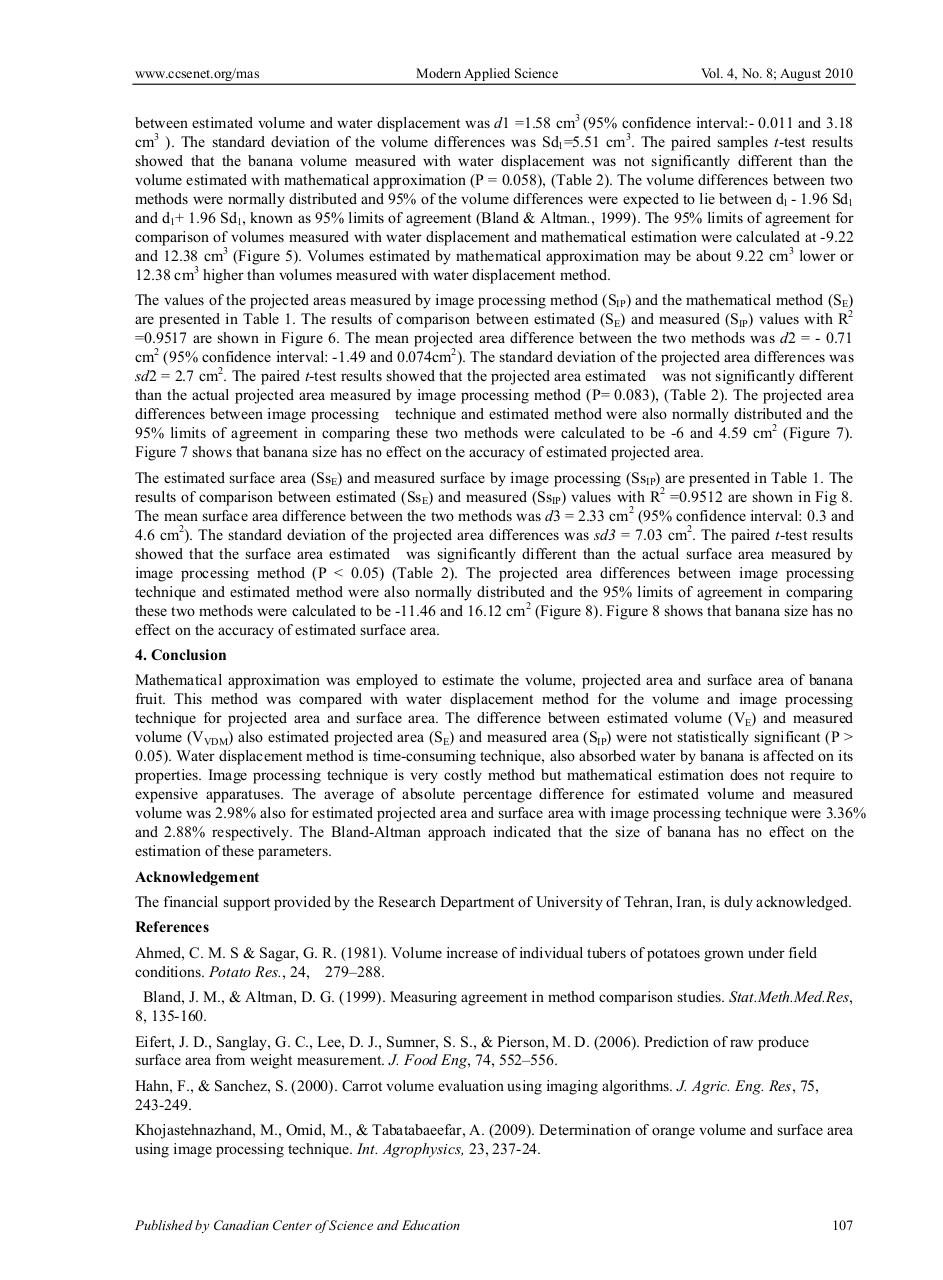# 5528.pdfPage 1 2 3 4 5 6 7 8 9 10

#### Text preview

www.ccsenet.org/mas

Modern Applied Science

Vol. 4, No. 8; August 2010

between estimated volume and water displacement was d1 =1.58 cm3 (95% confidence interval:- 0.011 and 3.18
cm3 ). The standard deviation of the volume differences was Sd1=5.51 cm3. The paired samples t-test results
showed that the banana volume measured with water displacement was not significantly different than the
volume estimated with mathematical approximation (P = 0.058), (Table 2). The volume differences between two
methods were normally distributed and 95% of the volume differences were expected to lie between d1 - 1.96 Sd1
and d1+ 1.96 Sd1, known as 95% limits of agreement (Bland &amp; Altman., 1999). The 95% limits of agreement for
comparison of volumes measured with water displacement and mathematical estimation were calculated at -9.22
and 12.38 cm3 (Figure 5). Volumes estimated by mathematical approximation may be about 9.22 cm3 lower or
12.38 cm3 higher than volumes measured with water displacement method.
The values of the projected areas measured by image processing method (SIP) and the mathematical method (SE)
are presented in Table 1. The results of comparison between estimated (SE) and measured (SIP) values with R2
=0.9517 are shown in Figure 6. The mean projected area difference between the two methods was d2 = - 0.71
cm2 (95% confidence interval: -1.49 and 0.074cm2). The standard deviation of the projected area differences was
sd2 = 2.7 cm2. The paired t-test results showed that the projected area estimated was not significantly different
than the actual projected area measured by image processing method (P= 0.083), (Table 2). The projected area
differences between image processing technique and estimated method were also normally distributed and the
95% limits of agreement in comparing these two methods were calculated to be -6 and 4.59 cm2 (Figure 7).
Figure 7 shows that banana size has no effect on the accuracy of estimated projected area.
The estimated surface area (SsE) and measured surface by image processing (SsIP) are presented in Table 1. The
results of comparison between estimated (SsE) and measured (SsIP) values with R2 =0.9512 are shown in Fig 8.
The mean surface area difference between the two methods was d3 = 2.33 cm2 (95% confidence interval: 0.3 and
4.6 cm2). The standard deviation of the projected area differences was sd3 = 7.03 cm2. The paired t-test results
showed that the surface area estimated was significantly different than the actual surface area measured by
image processing method (P &lt; 0.05) (Table 2). The projected area differences between image processing
technique and estimated method were also normally distributed and the 95% limits of agreement in comparing
these two methods were calculated to be -11.46 and 16.12 cm2 (Figure 8). Figure 8 shows that banana size has no
effect on the accuracy of estimated surface area.
4. Conclusion
Mathematical approximation was employed to estimate the volume, projected area and surface area of banana
fruit. This method was compared with water displacement method for the volume and image processing
technique for projected area and surface area. The difference between estimated volume (VE) and measured
volume (VVDM) also estimated projected area (SE) and measured area (SIP) were not statistically significant (P &gt;
0.05). Water displacement method is time-consuming technique, also absorbed water by banana is affected on its
properties. Image processing technique is very costly method but mathematical estimation does not require to
expensive apparatuses. The average of absolute percentage difference for estimated volume and measured
volume was 2.98% also for estimated projected area and surface area with image processing technique were 3.36%
and 2.88% respectively. The Bland-Altman approach indicated that the size of banana has no effect on the
estimation of these parameters.
Acknowledgement
The financial support provided by the Research Department of University of Tehran, Iran, is duly acknowledged.
References
Ahmed, C. M. S &amp; Sagar, G. R. (1981). Volume increase of individual tubers of potatoes grown under field
conditions. Potato Res., 24, 279–288.
Bland, J. M., &amp; Altman, D. G. (1999). Measuring agreement in method comparison studies. Stat.Meth.Med.Res,
8, 135-160.
Eifert, J. D., Sanglay, G. C., Lee, D. J., Sumner, S. S., &amp; Pierson, M. D. (2006). Prediction of raw produce
surface area from weight measurement. J. Food Eng, 74, 552–556.
Hahn, F., &amp; Sanchez, S. (2000). Carrot volume evaluation using imaging algorithms. J. Agric. Eng. Res, 75,
243-249.
Khojastehnazhand, M., Omid, M., &amp; Tabatabaeefar, A. (2009). Determination of orange volume and surface area
using image processing technique. Int. Agrophysics, 23, 237-24.